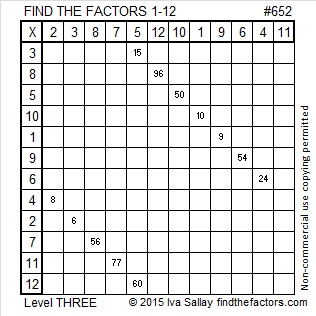# 652 and Level 3

652 has 6 factors. 6 is a perfect number because all of its smaller factors, 1, 2, and 3, add up to its largest factor, 6.

The factors of 652 are 1, 2, 4, 163, and 326. The sum of those factors is 496, another perfect number. Note that all of 496’s smaller factors, 1, 2, 4, 8, 16, 31, 62, 124, and 248, add up to 496, its largest factor.

OEIS.org states that 652 is the only known non-perfect number that produces a perfect number in both of those situations.Print the puzzles or type the solution on this excel file: 12 Factors 2015-10-19

—————————————————————————————————

• 652 is a composite number.
• Prime factorization: 652 = 2 x 2 x 163, which can be written 652 = (2^2) x 163
• The exponents in the prime factorization are 2 and 1. Adding one to each and multiplying we get (2 + 1)(1 + 1) = 3 x 2  = 6. Therefore 652 has exactly 6 factors.
• Factors of 652: 1, 2, 4, 163, 326, 652
• Factor pairs: 652 = 1 x 652, 2 x 326, or 4 x 163
• Taking the factor pair with the largest square number factor, we get √652 = (√4)(√163) = 2√163 ≈ 25.53429.—————————————————————————————————

A Logical Approach to solve a FIND THE FACTORS puzzle: Find the column or row with two clues and find their common factor. (None of the factors are greater than 12.)  Write the corresponding factors in the factor column (1st column) and factor row (top row).  Because this is a level three puzzle, you have now written a factor at the top of the factor column. Continue to work from the top of the factor column to the bottom, finding factors and filling in the factor column and the factor row one cell at a time as you go.This site uses Akismet to reduce spam. Learn how your comment data is processed.